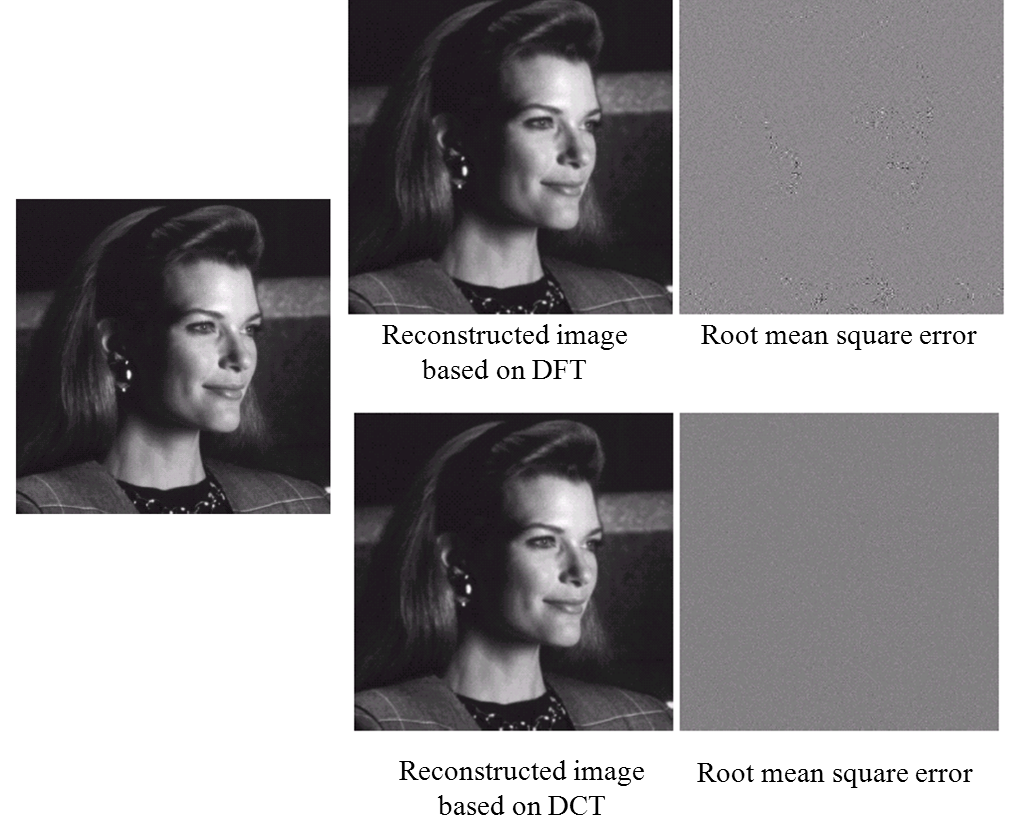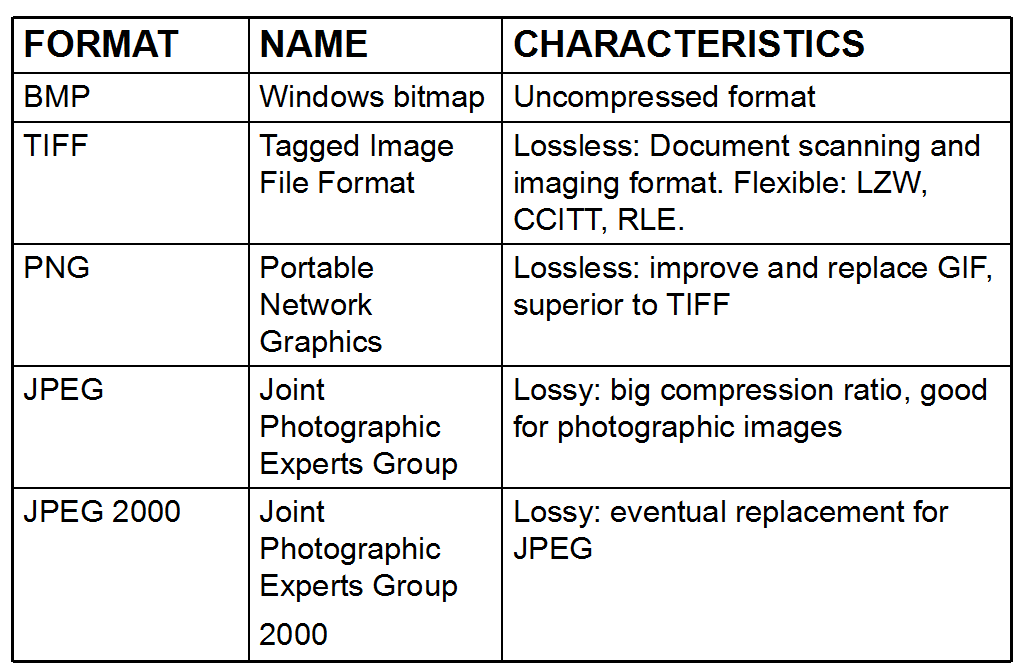1. $n_2 >> n_1$，压缩很失败，$R_D = -\infty,C_R = 0$，说明冗余过多，实际上只要$n_2 > n_1$，那么压缩就失败了,$R_D<0,C_R < 1$。
2. $n_2 = n_1$，相当于没有压缩，$R_D = 0, C_R = 1$。
3. $n_2 << n_1$，压缩成功，$R_D = 1,C_R = \infty$，实际上只要$n_2< n_1$，压缩就有效果，这时候$R_D > 0,C_R > 1$。

1. 编码冗余，指的是编码上不需要这么多的字长。
2. 像素间的冗余，比如相邻像素可能是一样的或者近似，有某种规律，可以用更简单的方式表示，这就是像素之间的冗余。
3. 视觉冗余，人的视觉没有太高的敏感度，比如颜色太相近人眼无法区分，或者图片略缩图比较小，不需要太多的灰度值来表示，又或者人眼对对比度光照等等更敏感，可以适当加大颜色的压缩比例等等。

### 编码冗余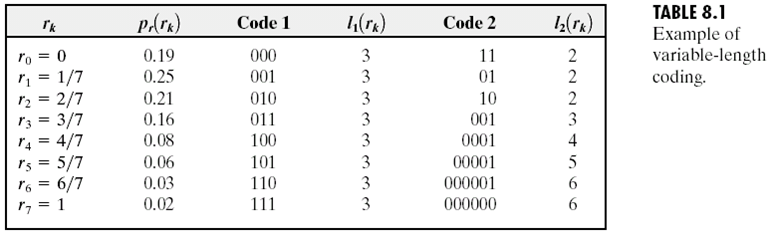### 像素间的冗余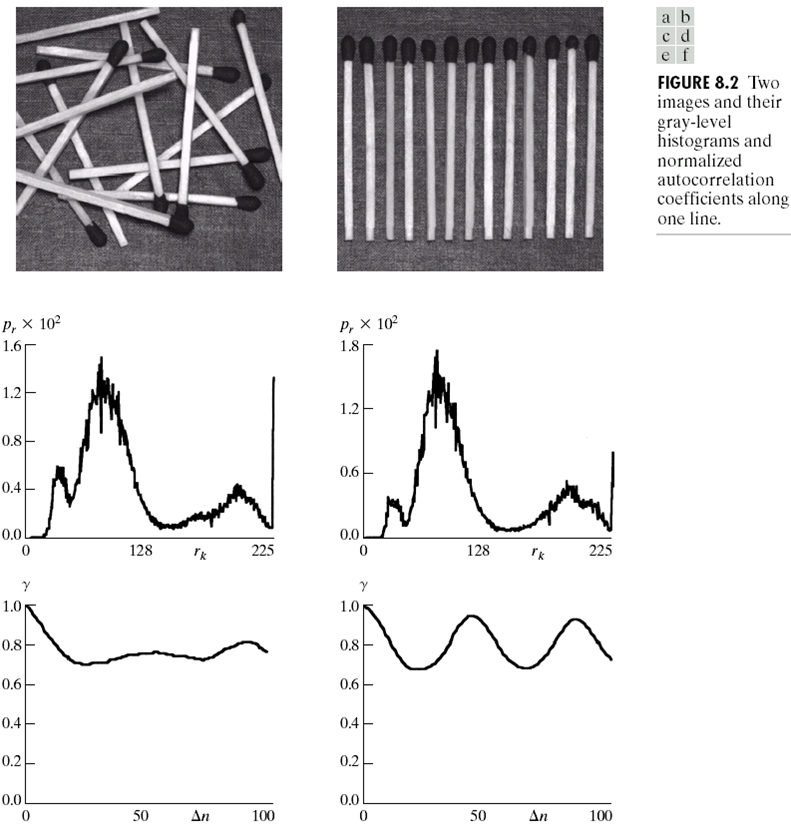$\Delta n$是像素间的距离，可以理解为步长。我们对$\Delta n$内的像素进行另一种形式的压缩。

$g_{n}$表示像素值，而$w_{n}$表示像素值连续出现的长度。这个方法有点像字典压缩算法。下面是一个例子：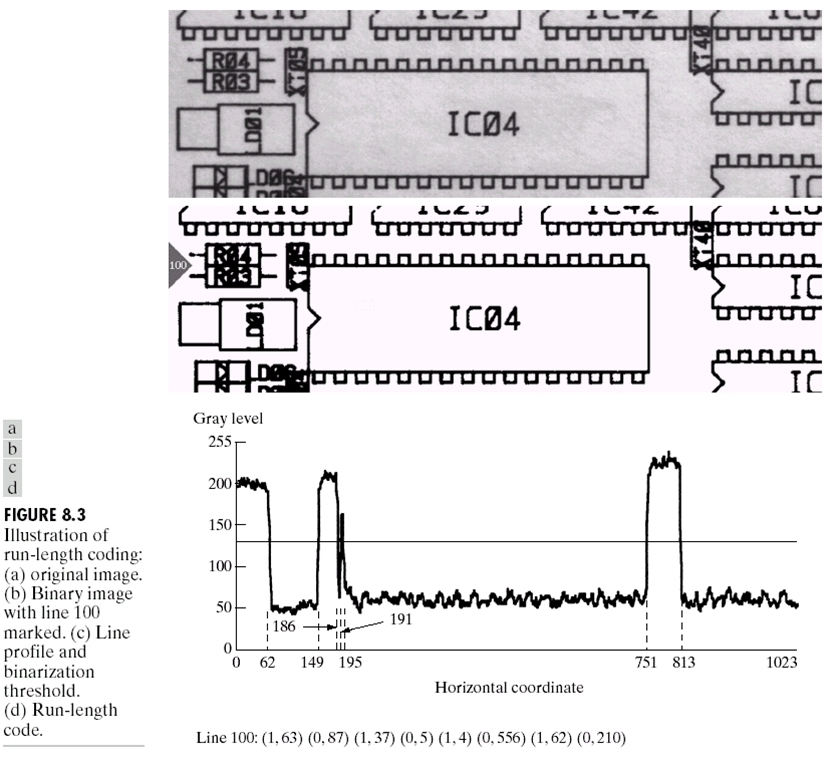### 视觉冗余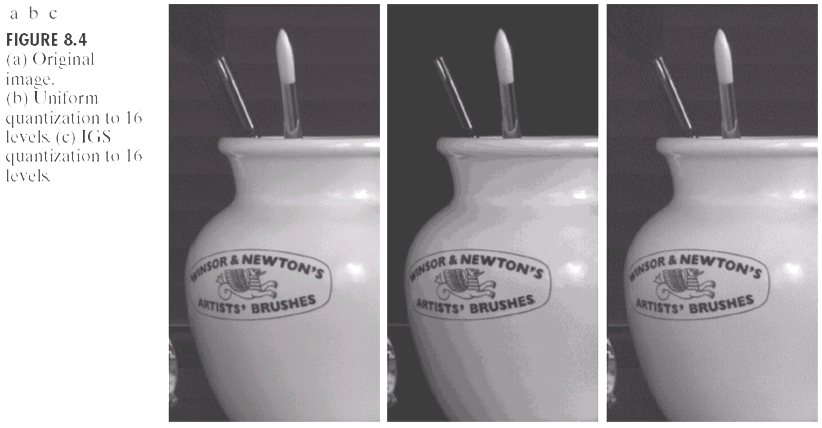• root mean square error:
• mean square signal-to-noise ratio: